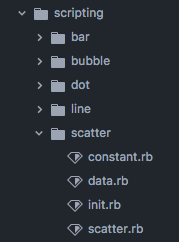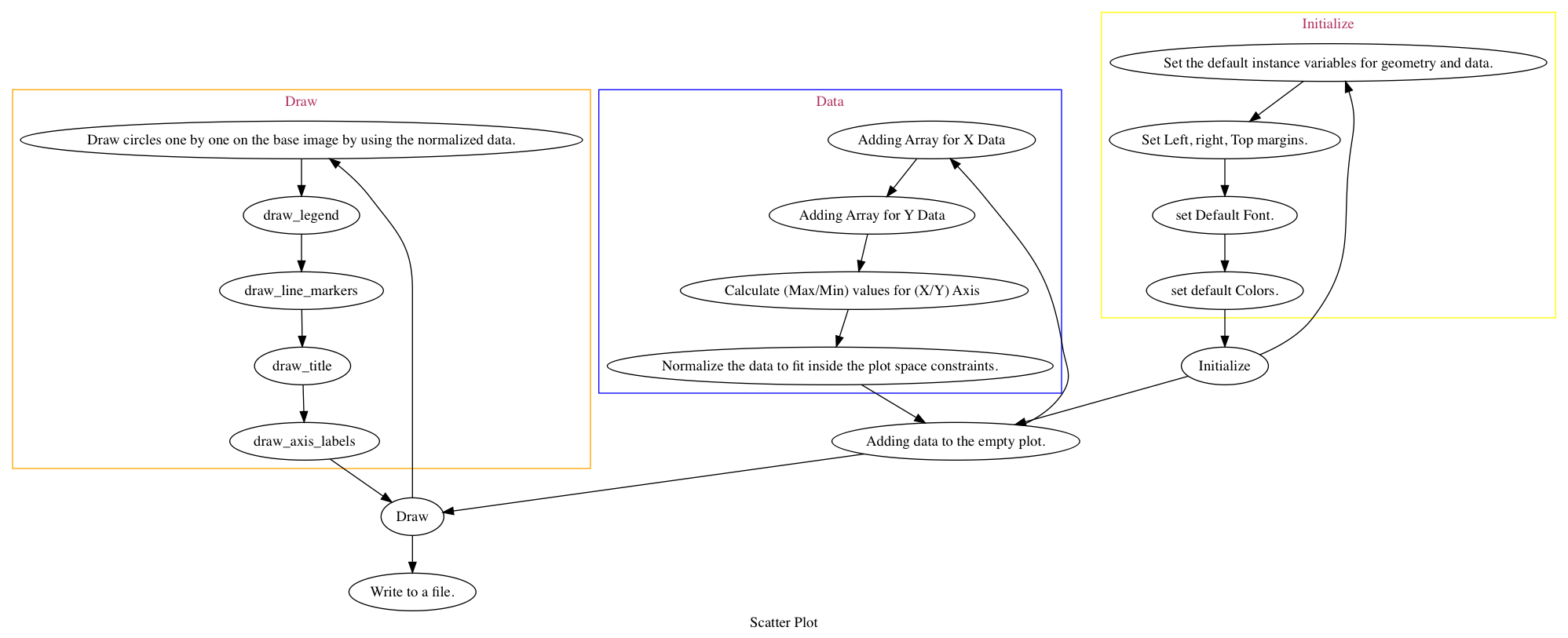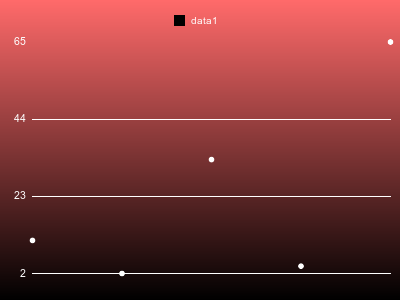# Scatter Plots

July 12, 2018

Let’s go through the scatter plot code base and see how we can use it for other ideas as well.
So currently the code base looks like this I have cleanly divided the scatter code base to 4 different files based on the applicability. All the files do exactly what their names suggest.Now let’s take a look at some example code to make scatter plot.

``````plot = Rubyplot::Scatter.new(400)
plot.data(:data1, [1, 2, 3, 4, 5], [11, 2, 33, 4, 65])
plot.write('spec/reference_images/scatterplot_testplot_1.png')
``````

This code is implemented internally in the library as ->Which makes an image asI hope this explains the whole concept of the construction of scatter plots. g